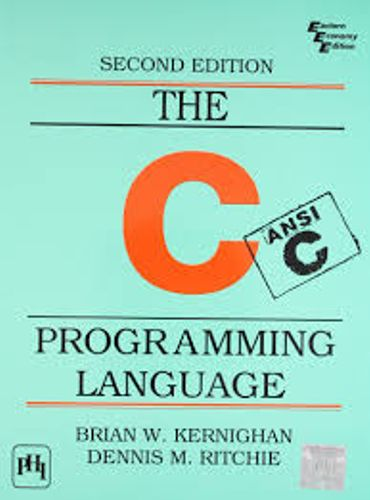# Arrays

Arrays are special variables which can hold more than one value under the same variable name, organised with an index. Arrays are defined using a very straightforward syntax:

```/* defines an array of 10 integers */
int numbers;
```

Accessing a number from the array is done using the same syntax. Notice that arrays in C are zero-based, which means that if we defined an array of size 10, then the array cells 0 through 9 (inclusive) are defined. `numbers` is not an actual value.

```int numbers;

/* populate the array */
numbers = 10;
numbers = 20;
numbers = 30;
numbers = 40;
numbers = 50;
numbers = 60;
numbers = 70;

/* print the 7th number from the array, which has an index of 6 */
printf("The 7th number in the array is %d", numbers);
```

Arrays can only have one type of variable, because they are implemented as a sequence of values in the computer’s memory. Because of that, accessing a specific array cell is very efficient.

## Home Work

• Define an int array which includes 10 numbers.

Click on image to Download C Programming Language Book :